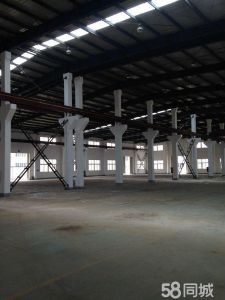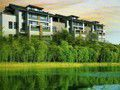﻿ 在线选址_中国招商投促网

基础设施
• 不限
• 五通一平
• 六通一平
• 七通一平
• 八通一平
• 九通一平
• 十通一平
• 十一通一平
主导产业
• 不限
• 电子信息
• 光伏机电
• 生物医药
• 能源材料
• 环保生态
• 航空航天
• 金融投资
• 食品加工
• 纺织服装
• 汽车产业
• 仓库物流
• 现代服务
• 石油化工
• 文化创意
• 包装印刷
• 装备制造
• 建材冶金
• 农副产品
• 机械加工
• 轻纺
• 高新技术
• 轨道交通
• 家具制造
• 电子商务
• 循环产业
• 其他
级       别
• 不限
• 国家级
• 省市级
• 市级以下
地       区
• 不限
• 北京市
• 天津市
• 河北省
• 山东省
• 江苏省
• 上海市
• 浙江省
• 福建省
• 江西省
• 安徽省
• 湖北省
• 湖南省
• 广东省
• 广西壮族自治区
• 海南省
• 重庆市
• 四川省
• 云南省
• 贵州省
• 辽宁省
• 吉林省
• 黑龙江省
• 山西省
• 陕西省
• 内蒙古自治区
• 宁夏回族自治区
• 甘肃省
• 青海省
• 新疆维吾尔自治区
• 西藏自治区

基础设施
• 不限
• 五通一平
• 六通一平
• 七通一平
• 八通一平
• 九通一平
• 十通一平
• 十一通一平
主导产业
• 不限
• 电子信息
• 光伏机电
• 生物医药
• 能源材料
• 环保生态
• 航空航天
• 金融投资
• 食品加工
• 纺织服装
• 汽车产业
• 仓库物流
• 现代服务
• 石油化工
• 文化创意
• 包装印刷
• 装备制造
• 建材冶金
• 农副产品
• 机械加工
• 轻纺
• 高新技术
• 轨道交通
• 家具制造
• 电子商务
• 循环产业
• 其他
地       区
• 不限
• 北京市
• 天津市
• 河北省
• 山东省
• 江苏省
• 上海市
• 浙江省
• 福建省
• 江西省
• 安徽省
• 湖北省
• 湖南省
• 广东省
• 广西壮族自治区
• 海南省
• 重庆市
• 四川省
• 云南省
• 贵州省
• 辽宁省
• 吉林省
• 黑龙江省
• 山西省
• 陕西省
• 内蒙古自治区
• 宁夏回族自治区
• 甘肃省
• 青海省
• 新疆维吾尔自治区
• 西藏自治区

业务意向
• 不限
• 出租
• 出售
• 求租
• 求购
建筑类别
• 不限
• 单层
• 双层
• 多层
• 独栋
建筑结构
• 不限
• 标准结构
• 排架结构
• 框架结构
• 砖混结构
• 钢铁结构
面       积
• 不限
• 500㎡以下
• 500㎡-1000㎡
• 1000㎡-1500㎡
• 1500㎡-2500㎡
• 2500㎡-4000㎡
• 4000㎡-6000㎡
• 6000㎡-10000㎡
• 10000㎡以上
地       区
• 不限
• 北京市
• 天津市
• 河北省
• 山东省
• 江苏省
• 上海市
• 浙江省
• 福建省
• 江西省
• 安徽省
• 湖北省
• 湖南省
• 广东省
• 广西壮族自治区
• 海南省
• 重庆市
• 四川省
• 云南省
• 贵州省
• 辽宁省
• 吉林省
• 黑龙江省
• 山西省
• 陕西省
• 内蒙古自治区
• 宁夏回族自治区
• 甘肃省
• 青海省
• 新疆维吾尔自治区
• 西藏自治区

业务意向
• 不限
• 出租
• 出售
• 求租
• 求购
土地性质
• 不限
• 综合用地
• 工业用地
• 商业用地
• 二手工业用地
面       积
• 不限
• 500㎡以下
• 500㎡-1000㎡
• 1000㎡-1500㎡
• 1500㎡-2500㎡
• 2500㎡-4000㎡
• 4000㎡-6000㎡
• 6000㎡-10000㎡
• 10000㎡以上
地       区
• 不限
• 北京市
• 天津市
• 河北省
• 山东省
• 江苏省
• 上海市
• 浙江省
• 福建省
• 江西省
• 安徽省
• 湖北省
• 湖南省
• 广东省
• 广西壮族自治区
• 海南省
• 重庆市
• 四川省
• 云南省
• 贵州省
• 辽宁省
• 吉林省
• 黑龙江省
• 山西省
• 陕西省
• 内蒙古自治区
• 宁夏回族自治区
• 甘肃省
• 青海省
• 新疆维吾尔自治区
• 西藏自治区

业务意向
• 不限
• 出租
• 出售
• 求租
• 求购
建筑类别
• 不限
• 单层
• 双层
• 多层
• 独栋
装修状况
• 不限
• 精装
• 简装
• 毛坯
面       积
• 不限
• 500㎡以下
• 500㎡-1000㎡
• 1000㎡-1500㎡
• 1500㎡-2500㎡
• 2500㎡-4000㎡
• 4000㎡-6000㎡
• 6000㎡-10000㎡
• 10000㎡以上
地       区
• 不限
• 北京市
• 天津市
• 河北省
• 山东省
• 江苏省
• 上海市
• 浙江省
• 福建省
• 江西省
• 安徽省
• 湖北省
• 湖南省
• 广东省
• 广西壮族自治区
• 海南省
• 重庆市
• 四川省
• 云南省
• 贵州省
• 辽宁省
• 吉林省
• 黑龙江省
• 山西省
• 陕西省
• 内蒙古自治区
• 宁夏回族自治区
• 甘肃省
• 青海省
• 新疆维吾尔自治区
• 西藏自治区

业务意向
• 不限
• 出租
• 出售
• 求租
• 求购
装修状况
• 不限
• 精装
• 简装
• 毛坯
租       金
• 不限
• 2000元以下
• 2000-5000元
• 5000-8000元
• 8000-10000元
• 10000-15000元
• 15000-20000元
• 20000元以上
面       积
• 不限
• 500㎡以下
• 500㎡-1000㎡
• 1000㎡-1500㎡
• 1500㎡-2500㎡
• 2500㎡-4000㎡
• 4000㎡-6000㎡
• 6000㎡-10000㎡
• 10000㎡以上
地       区
• 不限
• 北京市
• 天津市
• 河北省
• 山东省
• 江苏省
• 上海市
• 浙江省
• 福建省
• 江西省
• 安徽省
• 湖北省
• 湖南省
• 广东省
• 广西壮族自治区
• 海南省
• 重庆市
• 四川省
• 云南省
• 贵州省
• 辽宁省
• 吉林省
• 黑龙江省
• 山西省
• 陕西省
• 内蒙古自治区
• 宁夏回族自治区
• 甘肃省
• 青海省
• 新疆维吾尔自治区
• 西藏自治区###### (出租)出租京津科技谷400###### (出租)出租下朱庄独栋砖混结###### (出售)出售武清开发区50亩###### (出售)出售武清某乡镇级开发###### 中房富力城A座写字楼包装修低###### 好地段 精装修 带全部办公用

25200.00元/月###### 永和大厦A座200平米精装写

300000.00元/月###### 苏杭名苑###### 京能天下川###### 望都郡府